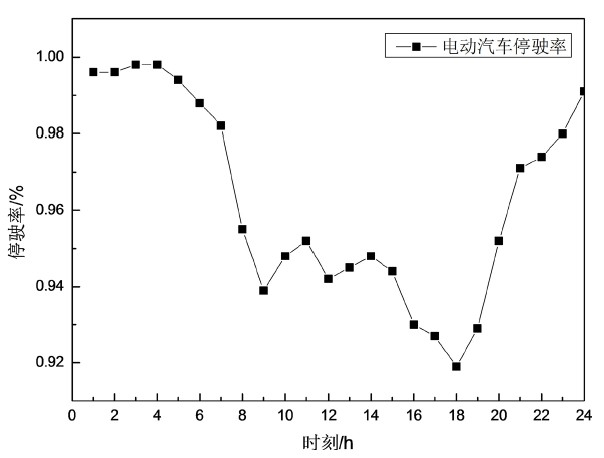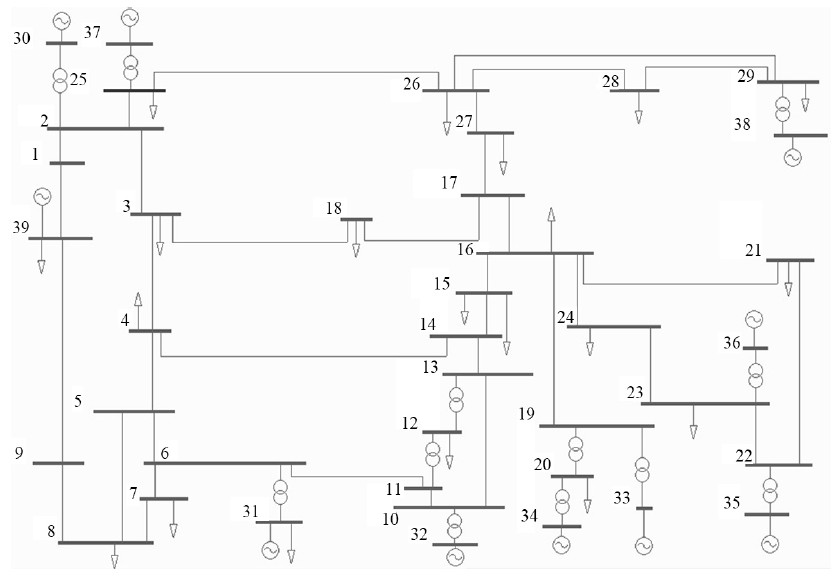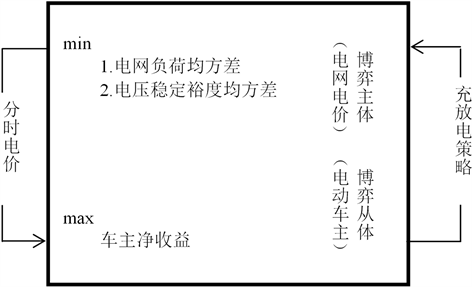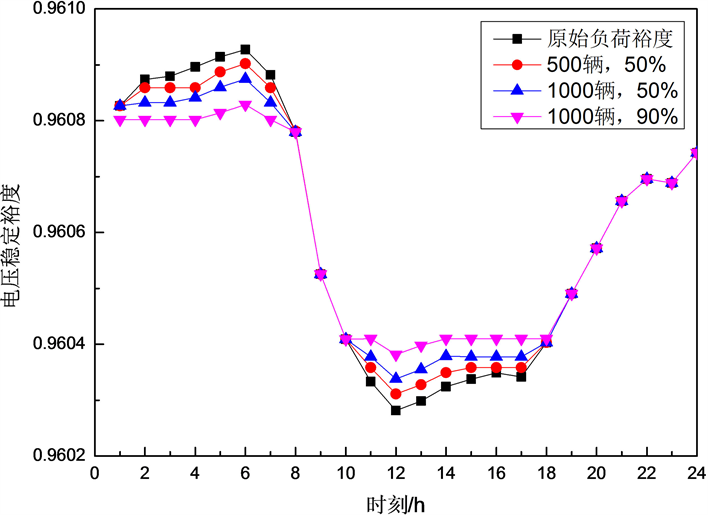# 基于主从博弈及分时电价引导的电动汽车充放电控制策略研究Study on Charge and Discharge Control Strategies of Electric Vehicles Based on Stackelberg Game and Time-of-Use Price Guidance

DOI: 10.12677/SG.2018.84034, PDF, HTML, XML, 下载: 573  浏览: 1,370

Abstract: With the increase in the number of electric vehicles entering the grid, how to maximize the inter-ests of both the grid and vehicle owners on the basis of meeting the stability of the electric power system is an urgent problem to be solved. Based on the Stackelberg game theory and the time-of-use price incentive mechanism, this paper establishes a time-average tariff that takes into account the load fluctuation rate of the grid and the owner's revenue, as well as the charging and discharging power optimization model of the electric vehicle. And under the comprehensive con-straints of grid load balancing, system static stability, and the capacity of electric vehicles that can be networked, the charging and discharging strategies and time-shared price of electric vehicles satisfying the maximization of the interests of both parties are determined. The results show that when the available capacity of electric vehicles accounts for 2.33% of the maximum load capacity, the Stackelberg game and the time-shared price guide strategy can reduce the peak load-to-valley load ratio of the power grid by 12.15% and the standard deviation of the voltage stability margin by 24.7%. The average daily income of vehicles reached 10.8 RMB, and good control effects were achieved.

1. 引言

2. 静态电压稳定性

2.1. 充放电负荷

$f\left(x\right)=\frac{1}{x\sigma \sqrt{2\text{π}}}\mathrm{exp}\left[-\frac{{\left(\mathrm{ln}x-\mu \right)}^{2}}{2{\sigma }^{2}}\right]$ (1)

$\text{SOC}=\left({\text{SOC}}_{0}-\frac{d}{{d}_{m}}\right)\ast 100%$ (2)Figure 1. The stopping rate of electric vehicles at each time

2.2. 电压稳定裕度

${V}_{\text{margin}}=\frac{{\lambda }_{\mathrm{max}}-1}{{\lambda }_{\mathrm{max}}}$ (3)

3. 主从博弈模型

3.1. 博弈模型及目标函数Figure 2. Simulation network system one-line diagramFigure 3. Flowchart of Stackelberg game process

${J}_{1}=\underset{t=1}{\overset{24}{\sum }}{\left({P}_{D,t}+{P}_{EV,t}-{P}_{avr}\right)}^{2}$ (4)

${P}_{avr}=\underset{t=1}{\overset{24}{\sum }}\left({P}_{D,t}+{P}_{EV,t}\right)/24$ (5)

${J}_{2}=\underset{t=1}{\overset{24}{\sum }}\text{ }{P}_{EV,t}{C}_{t}+{C}_{loss}$ (6)

${C}_{loss}=\underset{t=1}{\overset{24}{\sum }}\text{ }\mathrm{min}\left({P}_{EV,t},0\right){C}_{b}$ (7)

3.2. 约束条件

${C}_{ope}=\underset{t=1}{\overset{24}{\sum }}\left({P}_{D,t}+{P}_{EV,t}\right){C}_{t}$ (8)

${C}_{f}=\left({C}_{p}+{C}_{v}\right)/2$ (9)

$\underset{t=1}{\overset{24}{\sum }}\text{ }{P}_{EV,t}^{d}\le {P}_{total}^{d}$ (10)

$\underset{t=1}{\overset{24}{\sum }}\text{ }{P}_{EV,t}^{c}={P}_{total}^{c}$ (11)

$-{N}_{EV,t}^{\mathrm{max}}\ast {P}_{d}^{\mathrm{max}}\le {P}_{EV,t}\le {N}_{EV,t}^{\mathrm{max}}\ast {P}_{c}^{\mathrm{max}}$ (12)

3.3. 求解算法

${J}_{2}\left({\gamma }_{1},{\gamma }_{2}\right)\ge {J}_{2\mathrm{max}}-\epsilon$ (13)

${J}_{1}^{*}=\underset{{\gamma }_{1}\in {\Gamma }_{1}}{\mathrm{min}}\underset{{\gamma }_{2}\in {\Gamma }_{2}\left({\gamma }_{1}\right)}{\mathrm{max}}{J}_{1}\left({\gamma }_{1},{\gamma }_{2}\right)$ (14)

${J}_{1}\left({\gamma }_{1}^{*},{\gamma }_{2}^{*}\right)=\underset{{\gamma }_{2}\in {\Gamma }_{2}\left({\gamma }_{1}^{*}\right)}{\mathrm{min}}{J}_{1}\left({\gamma }_{1}^{*},{\gamma }_{2}\right)$ (15)

4. 算例

V2G模式下，不同规模及响应率电动汽车入网，负荷曲线变化情况如图4所示。Figure 4. Load curves of power grid under different V2G modesFigure 5. Voltage stability margin of power grid under different V2G modesTable 1. Comparison of load characteristics of electric vehiclesTable 2. Comparison of voltage stability of electric vehicleTable 3. Time-of-use price before and after the gameTable 4. Car owner’s income under different V2G modes

5. 结语

1) 电动汽车响应V2G策略入网有利于负荷平抑与电压稳定性的提高。在可用电池容量低于3%最大负荷容量的情况下，电动汽车的有序充放电可使负荷峰谷差率降低12.15%，电压稳定裕度的标准差提高24.7%。

2) 主从博弈模型适用于电动汽车参与V2G的分时电价决策问题。执行电网与电动汽车车主充分博弈后的分时电价，能够实现在电网售电收益不变的前提下，显著提升电动车主的综合收益。

3) 随着电动汽车规模以及电池响应率的增加，谷段电价的降低以及峰段、平段电价的提高，更有利于电网负荷曲线的平抑、电压稳定裕度的提高以及车主收益的增加。

  高赐威, 张亮. 电动汽车充电对电网影响的综述[J]. 电网技术, 2011, 35(2): 127-131.  Boulanger, A.G. (2011) Vehicle Electrification: Status and Issues. Proceedings of the IEEE, 99, 1116-1138. https://doi.org/10.1109/JPROC.2011.2112750  陈德树, 段献忠, 何仰赞. 电力系统电压稳定性的研究现状[J]. 电网技术, 1995, 19(4): 20-24.  余娟, 李文沉, 颜伟. 静态电压稳定风险评估[J]. 中国电机工程学报, 2009, 29(28): 40-46.  吴恒, 李升, 丁道剑. 电动汽车充电对静态电压稳定裕度影响的研究[J]. 南京工程学院学报(自然科学版), 2017, 15(2): 56-62.  郑颖, 孙近文, 张冲, 等. 考虑电动汽车接入的配电系统静态电压稳定裕度研究[J]. 电工技术学报, 2014, 29(8): 20-26.  徐从启, 高瑞林, 贾桂芝, 等. 考虑分时电价电动汽车负荷的微电网优化调度[J]. 电气工程学报, 2017, 12(11): 12-20.  魏大钧, 张承慧, 孙波, 等. 基于分时电价的电动汽车充放电多目标优化调度[J]. 电网技术, 2014, 38(11): 2972-2977.  徐智威, 胡泽春, 宋永华, 等. 基于动态分时电价的电动汽车充电站有序充电策略[J]. 中国电机工程学报, 2014, 34(22): 3638-3646.  Pantos, M. (2012) Exploitation of Electric-Drive Vehicles in Electricity Markets. IEEE Transactions on Power Systems, 27, 682-694. https://doi.org/10.1109/TPWRS.2011.2172005  Rotering, N. and Ilic, M. (2011) Optimal Charge Control of Plug-In Hybrid Electric Vehicles in Deregulated Electricity Markets. IEEE Transactions on Power Systems, 26, 1021-1029. https://doi.org/10.1109/TPWRS.2010.2086083  Rajaraman, R., Sarlashkar, J.V. and Alvarado, F.L. (1997) The Effect of Demand Elasticity on Security Prices for the PoolCo and Multi-Lateral Contract Models. IEEE Transactions on Power Systems, 12, 1177-1184. https://doi.org/10.1109/59.630459  杨国清, 付菁, 王德意, 等. 基于一主三从博弈的风–水–气区域电力系统调度研究[J]. 电网技术, 2018, 42(2): 495-502.  Li, Z.M., Li, W.B., Wang, M.Q., et al. (2017) Stackelberg Game Model of Wind Farm and Electric Vehicle Battery Switch Station. IOP Conference Series: Materials Science and Engineering, 199, 1-9.  杨国清, 王亚萍, 王德意, 等. 博弈论在光伏并网鲁棒优化调度问题中的应用[J]. 电力系统及其自动化学报, 2016, 28(8): 129-134.  魏韡, 陈玥, 刘锋, 等. 基于主从博弈的智能小区代理商定价策略及电动汽车充电管理[J]. 电网技术, 2015, 39(4): 939-945.  项顶, 宋永华, 胡泽春, 等. 电动汽车参与V2G的最优峰谷电价研究[J]. 中国电机工程学报, 2013, 33(31): 15-25.  翁国庆, 张有兵, 戚军, 等. 多类型电动汽车电池集群参与微网储能的V2G可用容量评估[J]. 电工技术学报, 2014, 29(8): 36-45.  刘利兵, 刘天琪, 张涛, 等. 计及电池动态损耗的电动汽车有序充放电策略优化[J]. 电力系统自动化, 2016, 40(5): 83-90.  Mozafar, M.R., Amini, M.H. and Moradi, M.H. (2018) Innovative Appraisement of Smart Grid Operation Considering Large-Scale Integration of Electric Vehicles Enabling V2G and G2V Systems. Electric Power Systems Research, 154, 245-256. https://doi.org/10.1016/j.epsr.2017.08.024